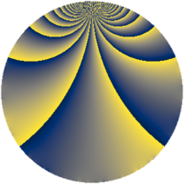# Properties

 Label 567.2.gLevel $567$ Weight $2$ Character orbit 567.g Rep. character $\chi_{567}(109,\cdot)$ Character field $\Q(\zeta_{3})$ Dimension $60$ Newform subspaces $12$ Sturm bound $144$ Trace bound $7$

# Related objects

## Defining parameters

 Level: $$N$$ $$=$$ $$567 = 3^{4} \cdot 7$$ Weight: $$k$$ $$=$$ $$2$$ Character orbit: $$[\chi]$$ $$=$$ 567.g (of order $$3$$ and degree $$2$$) Character conductor: $$\operatorname{cond}(\chi)$$ $$=$$ $$63$$ Character field: $$\Q(\zeta_{3})$$ Newform subspaces: $$12$$ Sturm bound: $$144$$ Trace bound: $$7$$ Distinguishing $$T_p$$: $$2$$, $$13$$

## Dimensions

The following table gives the dimensions of various subspaces of $$M_{2}(567, [\chi])$$.

Total New Old
Modular forms 168 68 100
Cusp forms 120 60 60
Eisenstein series 48 8 40

## Trace form

 $$60q - 30q^{4} - 3q^{7} + O(q^{10})$$ $$60q - 30q^{4} - 3q^{7} + 6q^{10} + 9q^{13} - 30q^{16} - 12q^{19} + 48q^{25} - 12q^{28} + 18q^{31} + 3q^{37} + 24q^{43} - 6q^{46} - 27q^{49} - 36q^{52} - 48q^{55} - 48q^{58} - 15q^{61} + 48q^{64} - 3q^{67} - 30q^{70} - 48q^{76} + 45q^{79} - 6q^{82} + 6q^{85} + 36q^{88} - 51q^{91} + 30q^{94} + 9q^{97} + O(q^{100})$$

## Decomposition of $$S_{2}^{\mathrm{new}}(567, [\chi])$$ into newform subspaces

Label Dim. $$A$$ Field CM Traces $q$-expansion
$$a_2$$ $$a_3$$ $$a_5$$ $$a_7$$
567.2.g.a $$2$$ $$4.528$$ $$\Q(\sqrt{-3})$$ None $$-2$$ $$0$$ $$-4$$ $$1$$ $$q+(-2+2\zeta_{6})q^{2}-2\zeta_{6}q^{4}-2q^{5}+\cdots$$
567.2.g.b $$2$$ $$4.528$$ $$\Q(\sqrt{-3})$$ None $$-1$$ $$0$$ $$-8$$ $$1$$ $$q+(-1+\zeta_{6})q^{2}+\zeta_{6}q^{4}-4q^{5}+(2+\cdots)q^{7}+\cdots$$
567.2.g.c $$2$$ $$4.528$$ $$\Q(\sqrt{-3})$$ $$\Q(\sqrt{-3})$$ $$0$$ $$0$$ $$0$$ $$-4$$ $$q+2\zeta_{6}q^{4}+(-3+2\zeta_{6})q^{7}+(-2+2\zeta_{6})q^{13}+\cdots$$
567.2.g.d $$2$$ $$4.528$$ $$\Q(\sqrt{-3})$$ $$\Q(\sqrt{-3})$$ $$0$$ $$0$$ $$0$$ $$5$$ $$q+2\zeta_{6}q^{4}+(3-\zeta_{6})q^{7}+(7-7\zeta_{6})q^{13}+\cdots$$
567.2.g.e $$2$$ $$4.528$$ $$\Q(\sqrt{-3})$$ None $$1$$ $$0$$ $$8$$ $$1$$ $$q+(1-\zeta_{6})q^{2}+\zeta_{6}q^{4}+4q^{5}+(2-3\zeta_{6})q^{7}+\cdots$$
567.2.g.f $$2$$ $$4.528$$ $$\Q(\sqrt{-3})$$ None $$2$$ $$0$$ $$4$$ $$1$$ $$q+(2-2\zeta_{6})q^{2}-2\zeta_{6}q^{4}+2q^{5}+(-1+\cdots)q^{7}+\cdots$$
567.2.g.g $$4$$ $$4.528$$ $$\Q(\sqrt{-2}, \sqrt{-3})$$ None $$0$$ $$0$$ $$0$$ $$-8$$ $$q-\beta _{2}q^{2}+(-4+4\beta _{1})q^{4}+\beta _{3}q^{5}+\cdots$$
567.2.g.h $$6$$ $$4.528$$ 6.0.309123.1 None $$-2$$ $$0$$ $$2$$ $$2$$ $$q+(-\beta _{1}-\beta _{4}+\beta _{5})q^{2}+(-2+\beta _{2}+\cdots)q^{4}+\cdots$$
567.2.g.i $$6$$ $$4.528$$ 6.0.309123.1 None $$2$$ $$0$$ $$-2$$ $$2$$ $$q+(1-\beta _{4}+\beta _{5})q^{2}+(-\beta _{1}-\beta _{2}-\beta _{3}+\cdots)q^{4}+\cdots$$
567.2.g.j $$8$$ $$4.528$$ 8.0.1767277521.3 None $$-1$$ $$0$$ $$4$$ $$-2$$ $$q+(-\beta _{2}-\beta _{6})q^{2}+(-\beta _{5}+\beta _{7})q^{4}+\cdots$$
567.2.g.k $$8$$ $$4.528$$ 8.0.1767277521.3 None $$1$$ $$0$$ $$-4$$ $$-2$$ $$q+(\beta _{2}+\beta _{6})q^{2}+(-\beta _{5}+\beta _{7})q^{4}+(-1+\cdots)q^{5}+\cdots$$
567.2.g.l $$16$$ $$4.528$$ $$\mathbb{Q}[x]/(x^{16} - \cdots)$$ None $$0$$ $$0$$ $$0$$ $$0$$ $$q-\beta _{1}q^{2}+(-\beta _{5}-\beta _{7}-\beta _{8}-\beta _{12}+\cdots)q^{4}+\cdots$$

## Decomposition of $$S_{2}^{\mathrm{old}}(567, [\chi])$$ into lower level spaces

$$S_{2}^{\mathrm{old}}(567, [\chi]) \cong$$ $$S_{2}^{\mathrm{new}}(63, [\chi])$$$$^{\oplus 3}$$$$\oplus$$$$S_{2}^{\mathrm{new}}(189, [\chi])$$$$^{\oplus 2}$$